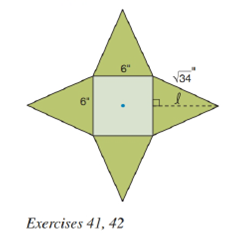Chapter 9.2, Problem 42E### Elementary Geometry for College St...

6th Edition
Daniel C. Alexander + 1 other
ISBN: 9781285195698

#### Solutions

Chapter
Section### Elementary Geometry for College St...

6th Edition
Daniel C. Alexander + 1 other
ISBN: 9781285195698
Textbook Problem
1 views

# Find the volume of the regular square pyramid that was formed in Exercise 41.To determine

To find:

The volume of the regular square pyramid.

Explanation

Given:

The four congruent isosceles triangles are folded upward it forms a regular square pyramid and the figure given below

From question 41, the slant height is 5" and base area is B=36in2.

Properties Used:

The volume V of a pyramid having a base area B and an altitude of length h is given by V=13Bh.

According to the Pythagorean theorem, in a right-angled triangle

hypotenuse2=base2+perpendicular2.

The area of the square is side2.

Approach Used:

i) Calculate the apothem of a square base a=side2.

ii) Using the property l2=a2+h2, find the height or altitude h by substituting the values of l and a.

iii) Calculate the base area B of the square base formed.

iv) Substitute the values of area B and the height h in equation V=13Bh to find the value of volume V.

Calculation:

From the figure below,

considering one of the lateral faces which is an isosceles triangle

### Still sussing out bartleby?

Check out a sample textbook solution.

See a sample solution

#### The Solution to Your Study Problems

Bartleby provides explanations to thousands of textbook problems written by our experts, many with advanced degrees!

Get Started

#### In Exercises 516, evaluate the given quantity. log100,000

Finite Mathematics and Applied Calculus (MindTap Course List)

#### Evaluate limx0sin(3+x)2sin9x.

Single Variable Calculus: Early Transcendentals, Volume I

#### limx1x3x21x = _____. a) 1 b) 1 c) 0 d) does not exist

Study Guide for Stewart's Single Variable Calculus: Early Transcendentals, 8th